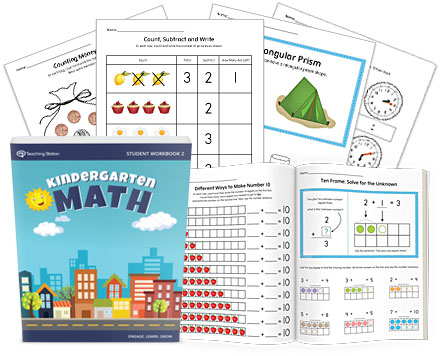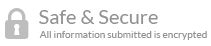• Teacher Approved

## Product ID: MTSMATH-KG-BK2

 Regular Price \$35.99
 Now \$15.75
Color:### Product Description

The Kindergarten Math Workbook Part 2 is specially designed to help students develop mathematical thinking by creating a strong foundation in number sense.

The workbook is packed with activities to promote critical thinking and analytical skills that create lifelong logic patterns to help comprehend advanced mathematical concepts in future grades.

Topics include composing and decomposing numbers, word problems, addition, subtraction, two and three-dimensional shapes, counting money, skip counting by 5s, time and calendar.

The Kindergarten Math Workbook Part 2 is part of the Complete Kindergarten Math Curriculum and MTS Math Program.

### Part 2 Major Skills and Concepts

• Learn to compose and decompose numbers within 10 (e.g., 4 + 3 = 7 and 7 = 4 + 3).
• Develop number sense by the use of numbers in different ways and situations.
• Make sense of word problems and develop the skill to solve for the unknown number.
• Learn and apply math rules to solve basic addition and subtraction problems.
• Understand and solve number sentences (e.g., 2 + 3 = 5 ) within the context of 10.
• Apply different techniques to comprehend, interpret, evaluate, and solve math problems.
• Learn to count by 5s.
• Learn and use tally marks to quickly count by 5s and record ongoing results.
• Identify and describe two-dimensional shapes including pentagon, hexagon, parallelogram, and trapezoid.
• Identify and decompose two-dimensional shapes.
• Develop a basic understanding of geometric terms such as side, vertex (corners) and parallel lines.
• Develop the conceptual understanding of subtraction as taking apart (or taking from) a number.
• Learn to subtract numbers within 10 using pictures, a ten-frame, and other visual counters.
• Recognize pennies, nickels, dimes, quarters and identify their values.
• Understand how to count money using coins.
• Recognize three-dimensional shapes including sphere, cube, cylinder, pyramid, cone, triangular prism, and rectangular prism.
• Understand the difference between two and three-dimensional shapes.
• Understand how time is measured, how many hours are in a day and how many minutes are in an hour.
• Read time using a digital and analog clock.
• Learn the days of the week, months of the year and their ordinal sequence.

### Can I see a sample of the program lessons?

Yes, of course! Download free sample lessons from the Kindergarten Math Workbook Part 1.

There are no reviews yet.

Be the first to review the Learning the Alphabet product.

 *Public Name This will be the nickname shown on your review. *Email Your email will not be published. *Your Rating 1 2 3 4 5 Your Review

#### File Info

 Package: 2 Files Size: 401 Total Pages Format: PDF